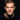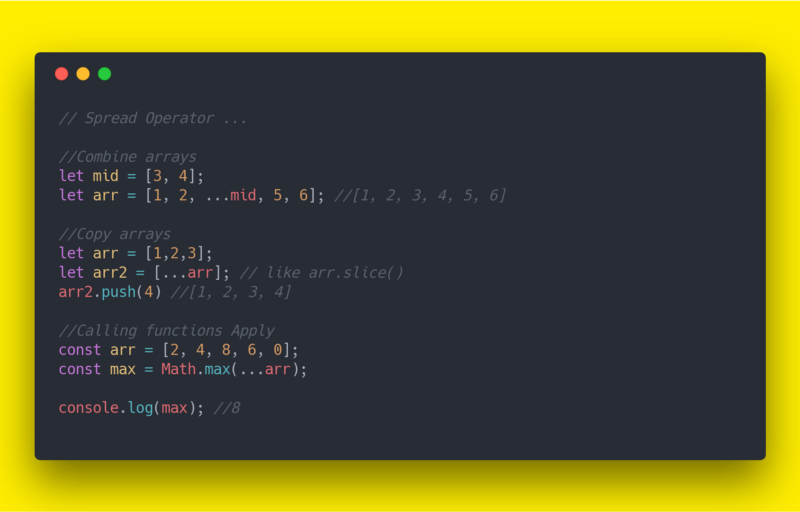# ES6 cheatsheet  —  Spread OperatorSerban Mihai / 20 October 2018The spread syntax is simply three dots: `...` It allows an iterable to expand in places where 0+ arguments are expected.

### Calling Functions without Apply:

``````function doStuff (x, y, z) { }
var args = [0, 1, 2];

// Call the function, passing args
doStuff.apply(null, args);

doStuff(...args);``````

``````const arr = [2, 4, 8, 6, 0];
const max = Math.max(...arr);

console.log(max); //8``````

Or another example using Math functions:

``````let mid = [3, 4];
let arr = [1, 2, ...mid, 5, 6]; //[1, 2, 3, 4, 5, 6]``````

### Combine arrays

``````let arr = [1,2,3];
let arr2 = [...arr]; // like arr.slice()
arr2.push(4)``````

You can find a more complete ES6 cheetsheet on my Github page.++= ❤️[Source code]